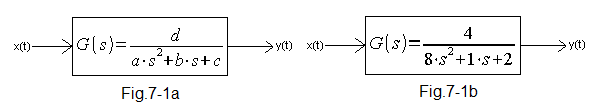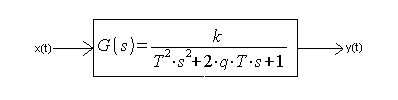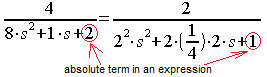### Chapter 7 What if we could do 2 bits shifts?

Chapter 7.1 Introduction
The real CRC size is 1or bytes. But what’s the other difference between this CRC you know until now and the real CRC? There are registers shifts, mainly 1 byte shifts. Transmission program sends byte after byte to the so-called UART electronic circuit and this circuit changes this bytes stream for bits stream. The accurate knowledge how to do it, isn’t necessary for programmer. This is UART job which was invented several dozen years before. It means, that program should predict- calculate the CRC state after 8 bits shifts. It springs to mind the program simulating 8 bits shifts from the chapter 5.2. This is time-consuming SIMPLE method. So it isn’t used in practice.There are used so-called table methods. They aren’t easy because they require big table size. 256×8 or 256×32 even!
So we start with 2 bits shifts. The small 4×8 tables are easier to understand. You will have no problems with 8 shifts in the next chapter.

Chapter 7.2     One byte CRC with 1 bit shift

##Fig. 7-1
One byte CRC with 1 bit shift    ANIMATION
This is a more real Chapter 5.2 version. There is 8 bit CRC register instead of 4 bit CRC. The message A is a little “more real” too. The message (in other words – the “dividend”) is 2 byte long instead of 1 byte. But remember that real message A may be thousands bytes long!
The factor=1 10010101 so the 8 lower digits, i.e. 10010101 named poly are in the table(1). Please note that used here CRC method is a table method too! This 2×8 table is quite normal!
The animation will convince you that the CRC is calculated bit after bit, i.e. SIMPLE Method. The next examples are the modifications as 2 bits shifts–>this chapter and 8 bits shifts–> next chapter. The message (A register) and poly are always the same–>10010101 so the calculated CRC values should be the same too. But it’s different in the Chapter 7.6 and I don’t know why? Help!!! I rely on dear readers help here.

Chapter 7.3 What’ s the CRC after two bits shift?
Chapter 7.3.1 Introduction
We know the CRC state after 1 bit shift. We can predict the state after 2 bit shifts then.
All possible initial CRC cominations i.e. 00, 01, 10 and 11 will be considered.

Chapter 7.3.2 Two bits shifts when 2 first CRC are 00Fig. 7-2
CRC with 2 first bits 00 ANIMATION
This case is banal. The bits abcdefgh will be shifted only, because BS=0 after all shift. CRC will be xor-ed with 00000000 and this operation doesn’t change the CRC.

Fig. 7-2a shows initial CRC state as 8 bits between red lines. CRC register is xor-ed with 00000000 values in the next 2 “zegar-clock” steps.
Fig. 7-2b It’s very easy to construct logical product with negations using xor operation. Remember this rule!
Fig. 7-2c The ultimate CRC as initial CRC value xor-ed by any value–> 00000000 here.
Conclusion:
Ultimate CRC=abcdefgh xor 00000000 when 2 initial CRC are 00.

Chapter 7.3.3 Two bits shifts when 2 first CRC are 01Fig. 7-3
CRC with 2 first bits 01   ANIMATIONThe CRC state calculation after 2 bit shift was banal  in the chapter 7.3.2. There were shifts only.
The CRC initial state is CRC=01abcdef now. The remaining 2 gh bits are waiting in A memory now.
There are 2 methods to predict the CRC state after 2 bits shifts.
1. Observe CRC animation. Sometimes shows up the canopies as negations over abcdefgh symbols when xor-ed. Try to smash it yourself.
2. Manual  arithmetic as CRC xor-ing with the shifted rows between red lines. Try to smash it yourself too.
This algorithm should be:
-Write 01abcdefgh. CRC=01abcdef. This is a initial CRC state as 8 bits between 2 red lines–>Fig.7-3a.
The next BS=0 because the first CRC bit=0.
-Append shifted 00000000 over initial state because BS=0 now. The new CRC state is a xor of 2 rows between red lines. Is this the same as in animation? Check it for your own satisfaction. The next BS=1 as a xor of the first column to the left of the red line.
-Append shifted 10010101 over 2 row because BS=1 now.
The new and ultimate CRC state is a xor of the 3 rows between red lines i.e. CRC=abcdefgh xor 10010101 as in Fig. 7-3b.
Note that there are “canopies” over symbols a, d, f and h. These values are negated.
Conclusion:
Ultimate CRC=abcdefgh xor 10010101 when 2 initial CRC are 01.
How does interpret the value 10010101 after xor symbol? This is xor of the 2 rows over abcdefgh in last step.

Chapter 7.3.4 Two bits shifts when 2 first CRC are 10Fig. 7-4
CRC with 2 first bits 10 ANIMATION
Conclusion:
Ultimate CRC=abcdefgh xor 10111111 when 2 initial CRC are 10.

Chapter 7.3.5 Two bits shifts when 2 first CRC are 11Fig. 7-5
CRC with 2 first bits 11    ANIMATION
Conclusion:
Ultimate CRC=abcdefgh xor 00101010 when 2 initial CRC are 11.

Chapter 7.3.6 Table as a summary of chapter 7.3
When 2 first CRC bits are:
– 00 then after 2 shifts CRC=CRC xor 00000000
– 01
then after 2 shifts CRC=CRC xor 10010101
– 10
then after 2 shifts CRC=CRC xor 10111111
– 11 then after 2 shifts CRC=CRC xor 00101010
This knowledge is presented as a 4×8 table –> Fig. 7-6

Chapter 7.4 Direct table method for 2 bits shiftsFig. 7-6
Direct
table method for 2 bits shifts  ANIMATION
2
bytes message – is shifting left through CRC and bits jumps are used here. Compare the 4×8 table with the Chapter 7.3.6.
I hope that animation says more than thousands words.

Chapter 7.5 The “Left” table method with double xor for 2 bits shifts
Why “double xor”? You will know in a while.Fig. 7-7
“Left”
table method with double xor for 2 bits shifts  ANIMATION
The 2 initial A and CRC bits are  xor-es first and shifed to BS then. It’s more smart and concrete than direct table method–>chapter 7.5. The B register doesn’t exists and there isn’t “empty flow of zeroes” at the start. The tables were formed acc. this same poly 10010101 so  all the  chapter 7.2, 7.4, 7.5  and 7.6 ultimate CRC values  should be the same CRC=11100111!

Chapter 7.6 The “Right” table method with double xor for 2 bits shiftsFig. 7-8
“Right” table method with double xor for 2 bits shifts  ANIMATION
What’s the matter? The previous “Left” table method was correct. Why does improve the good method? Let’s return to Chapter 7.1 for a moment. All the leaving out bits are shifted trough the so-called UART circuit. There are many UARTs which treats the byte=00000001 as 128, not 1 as for normal people! The right bit is more older. How do we cure this problem?  We can swing around (reverse) the bites in the byte and use “Left” method  for example. But it’s time consuming. The “Right” table method doesn’t need bits reversing. But all others will be reverse.
I remind that “quarter-bytes” and “bytes” in this chapter simulate the “bytes” and “four bytes” in the next 8 chapter. The incoming “quarter-bytes” are treat as a normal here. I.e.  left bit is older than a right.
So the “left” outgoing first 4 “quarter-bytes” 10101001 (Fig. 7-7) and “right” outgoing 4 “quarter-bytes” 01101010 (Fig. 7-8) are treated by the receiver as this same 10101001 value! In other words. “Quarter-bytes” in A memory  are “swinged around”- (another word “reversed”) regarded  Fig. 7-7 but bits in the “quarter-bytesdoesn’t change.
The next 3 conditions must be fullfilled too:
1. All the table rows are reversed–>row 11111101 (Fig. 7-7) and 10111111 (Fig. 7-8) are reversed for example.
2. Initial CRC state is reversed. The state 00000000 will be not changed of course.
3. The ultimate CRC is reversed.
But I have mixed feelings here. The ultimate CRC=10010101 and is different from chapters 7.2, 7.4, 7.5. This is the original sin of this course! There is a bug somwhere. So treat the chapter 7.6 with some distance. I rely on my dear readers. Who will calculate right  CRC=10010101 and locate the mistake here?

Chapter. 7.7 CRC Software
Chapter 7.7.1 Introduction
The  message to send is in the A register.
The tables were formed as in the  chapter 7.4, but remember that the “right” table is some another than the “left” and “direct”. I propose the tables program creation for more ambitious readers. The certain pseudolanguage type will be used here.

Chapter 7.7.2 Direct table method for 2 bits shifts
BS CRC A B registers form common BS_CRC_A_B shift register.
Commands description:
Write message to CRC left – do 2 bits shifts left in  BS_CRC_A_B (as in the animation)
While A is not empty – start of the loop
CRC=CRC xor table(BS) CRC is xor-ed by the table value addressed by the BS

The program is very simple
B = 0
CRC=0
While A is not empty
{
Write message to CRC left
CRC
=CRC xor table(BS)
}
When the loop is finished (i.e. A is empty) CRC contains ultimate value  (calculated “remainder”)

Chapter 7.7.3 The “Left” table method with double xor for 2 bits shifts
The “zeroes” B register doesn’t exist here.
This program is some complicated than a previous-“direct”
CRC=0
While A is not empty
{
BS
=2 initial BS bits xor 2 initial BS bits
Shift 2 bits A left
Shift 2 bits CRC left
CRC
=CRC xor table(BS)
}
When the loop is finished (i.e. A is empty) CRC contains ultimate value  (calculated “remainder”)

Chapter 7.7.4 The “Right” table method with double xor for 2 bits shifts
Remember about tables and CRC notes in the Chapter 7.6. Especially-The “quarter-bytes in A register are reversed but 2 bites in “quarter-bytes aren’t! In that programmer doesn’t need to reverse “quarter-bytes when send out via UART!

CRC=0
While A is not empty
{
BS=2 initial BS bits
xor 2 initial BS bits
Shift 2 bits A right
Shift 2 bits CRC right
CRC
=CRC xor table(BS)
}
reverse CRC
When the loop is finished (i.e. A is empty) CRC contains ultimate value  (calculated “remainder”)